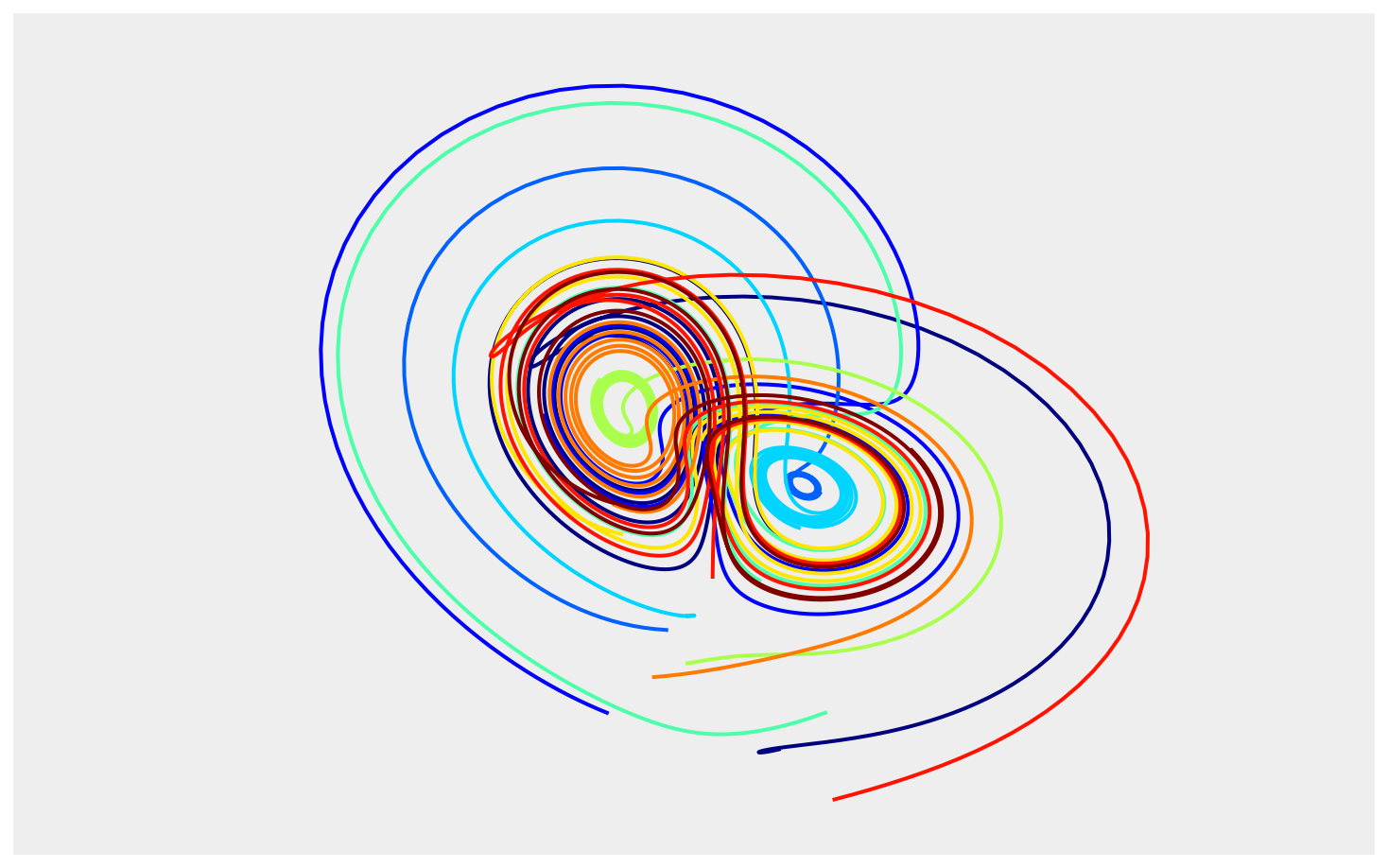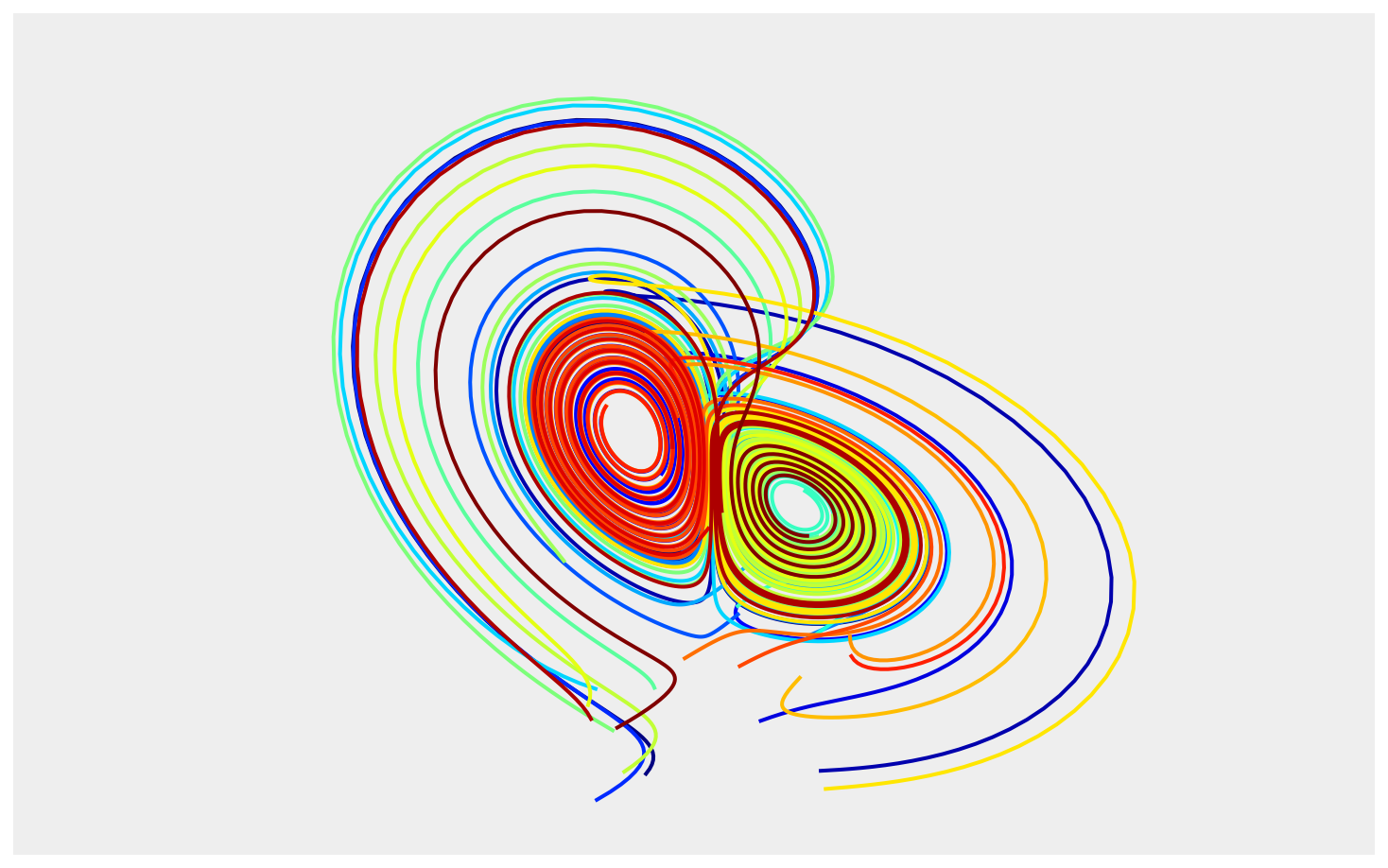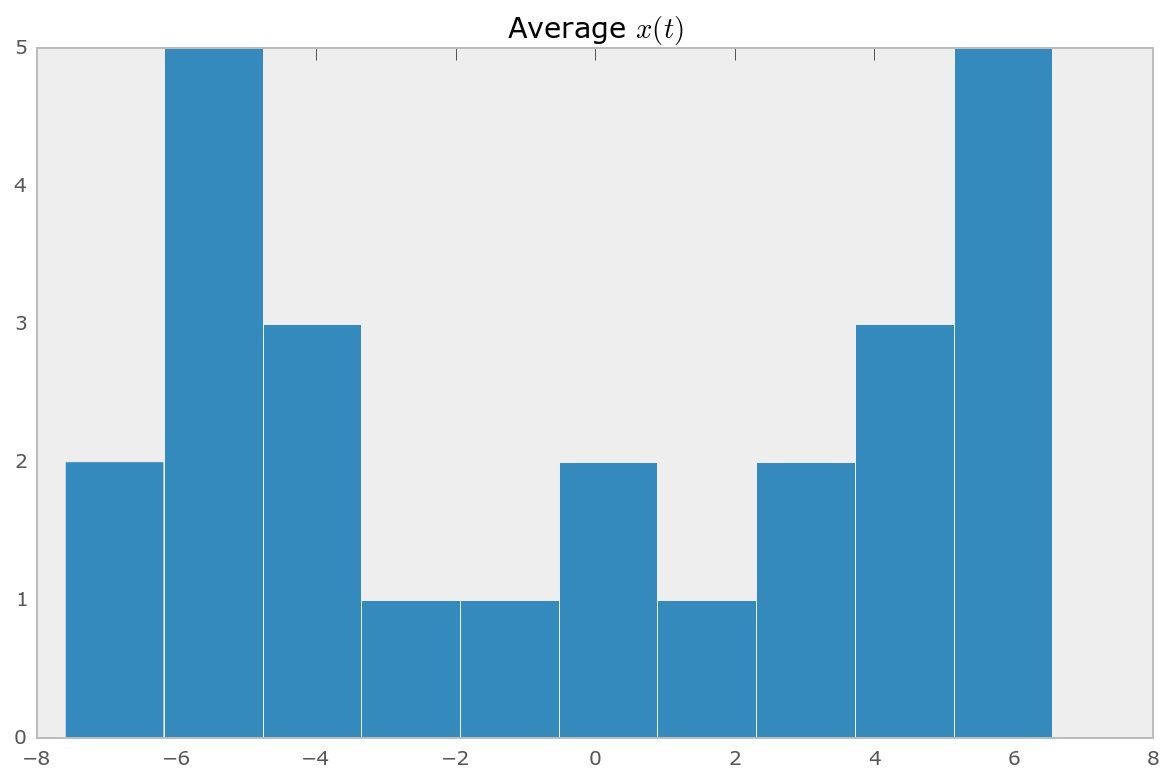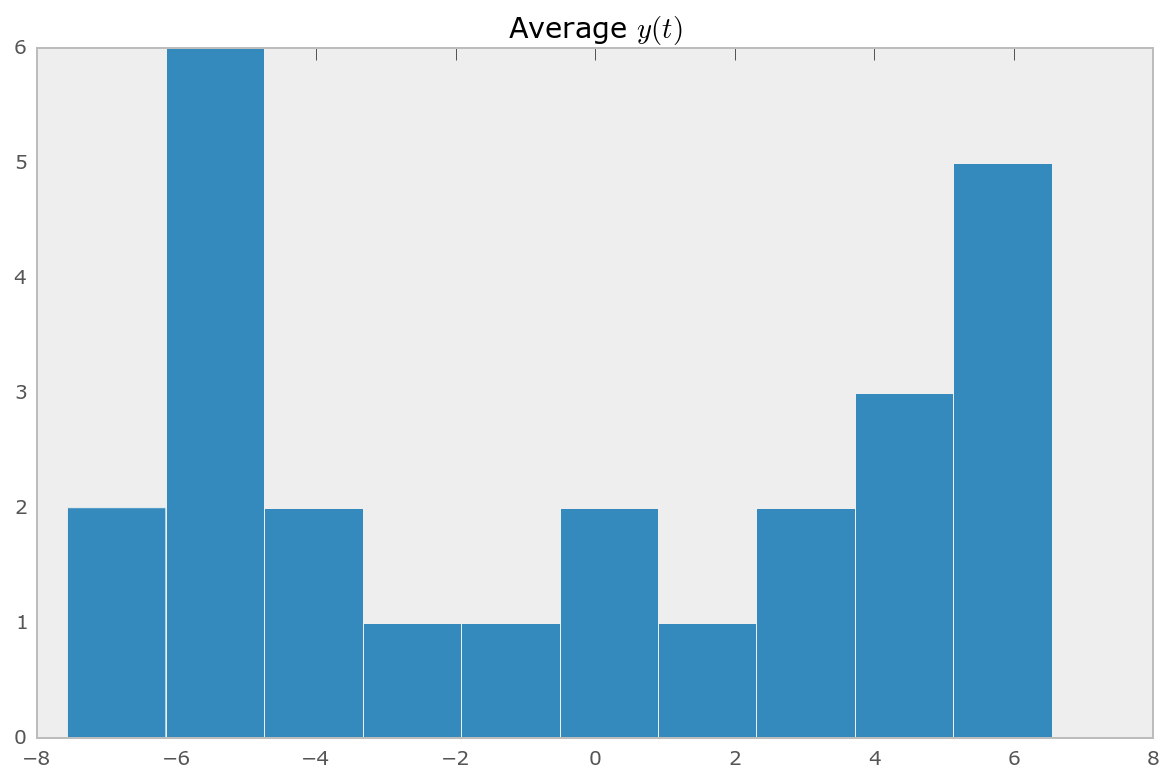# Exploring the Lorenz System of Differential Equations¶

In this Notebook we explore the Lorenz system of differential equations:

\begin{aligned} \dot{x} & = \sigma(y-x) \\ \dot{y} & = \rho x - y - xz \\ \dot{z} & = -\beta z + xy \end{aligned}

This is one of the classic systems in non-linear differential equations. It exhibits a range of different behaviors as the parameters ($\sigma$, $\beta$, $\rho$) are varied.

## Imports¶

First, we import the needed things from IPython, NumPy, Matplotlib and SciPy.

In :
%matplotlib inline

In :
from IPython.html.widgets import interact, interactive
from IPython.display import clear_output, display, HTML

In :
import numpy as np
from scipy import integrate

from matplotlib import pyplot as plt
from mpl_toolkits.mplot3d import Axes3D
from matplotlib.colors import cnames
from matplotlib import animation


## Computing the trajectories and plotting the result¶

We define a function that can integrate the differential equations numerically and then plot the solutions. This function has arguments that control the parameters of the differential equation ($\sigma$, $\beta$, $\rho$), the numerical integration (N, max_time) and the visualization (angle).

In :
def solve_lorenz(N=10, angle=0.0, max_time=4.0, sigma=10.0, beta=8./3, rho=28.0):

fig = plt.figure()
ax = fig.add_axes([0, 0, 1, 1], projection='3d')
ax.axis('off')

# prepare the axes limits
ax.set_xlim((-25, 25))
ax.set_ylim((-35, 35))
ax.set_zlim((5, 55))

def lorenz_deriv((x, y, z), t0, sigma=sigma, beta=beta, rho=rho):
"""Compute the time-derivative of a Lorentz system."""
return [sigma * (y - x), x * (rho - z) - y, x * y - beta * z]

# Choose random starting points, uniformly distributed from -15 to 15
np.random.seed(1)
x0 = -15 + 30 * np.random.random((N, 3))

# Solve for the trajectories
t = np.linspace(0, max_time, int(250*max_time))
x_t = np.asarray([integrate.odeint(lorenz_deriv, x0i, t)
for x0i in x0])

# choose a different color for each trajectory
colors = plt.cm.jet(np.linspace(0, 1, N))

for i in range(N):
x, y, z = x_t[i,:,:].T
lines = ax.plot(x, y, z, '-', c=colors[i])
plt.setp(lines, linewidth=2)

ax.view_init(30, angle)
plt.show()

return t, x_t


Let's call the function once to view the solutions. For this set of parameters, we see the trajectories swirling around two points, called attractors.

In :
t, x_t = solve_lorenz(angle=0, N=10)Using IPython's interactive function, we can explore how the trajectories behave as we change the various parameters.

In :
w = interactive(solve_lorenz, angle=(0.,360.), N=(0,50), sigma=(0.0,50.0), rho=(0.0,50.0))
display(w)The object returned by interactive is a Widget object and it has attributes that contain the current result and arguments:

In :
t, x_t = w.result

In :
w.kwargs

Out:
{u'N': 25,
u'angle': 180.0,
u'beta': 2.6666666666666665,
u'max_time': 4.0,
u'rho': 25.0,
u'sigma': 25.0}

After interacting with the system, we can take the result and perform further computations. In this case, we compute the average positions in $x$, $y$ and $z$.

In :
xyz_avg = x_t.mean(axis=1)

In :
xyz_avg.shape

Out:
(25, 3)

Creating histograms of the average positions (across different trajectories) show that on average the trajectories swirl about the attractors.

In :
plt.hist(xyz_avg[:,0])
plt.title('Average $x(t)$')

Out:
<matplotlib.text.Text at 0x10c0387d0>In :
plt.hist(xyz_avg[:,1])
plt.title('Average $y(t)$')

Out:
<matplotlib.text.Text at 0x10cadb610>